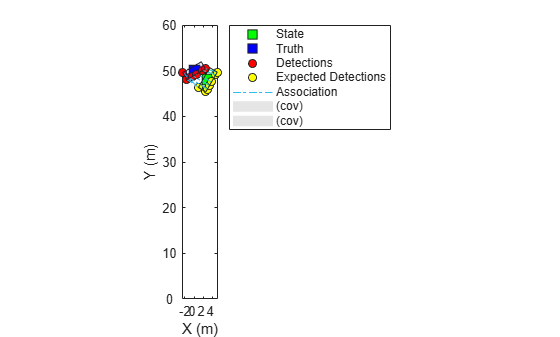# ctrectmeas

Constant turn-rate rectangular target measurement model

## Syntax

``measurements = ctrectmeas(states,detections)``

## Description

example

````measurements = ctrectmeas(states,detections)` returns the expected measurements from the current rectangular states and detections.```

## Examples

collapse all

Load detections and truth generated from a rectangular target.

`load('rectangularTargetDetections.mat','detections','truthState');`

Generate expected detections from the target's rectangular state and actual detections using `ctrectmeas`.

```tgtState = [3;48;0;60;0;5;1.9]; zExp = ctrectmeas(tgtState,detections);```

Set up visualization environment using `theaterPlot`.

```theaterP = theaterPlot; stateP = trackPlotter(theaterP,'DisplayName','State','MarkerFaceColor','g'); truthP = trackPlotter(theaterP,'DisplayName','Truth','MarkerFaceColor', 'b'); detP = detectionPlotter(theaterP,'DisplayName','Detections','MarkerFaceColor','r'); expDetP = detectionPlotter(theaterP,'DisplayName','Expected Detections','MarkerFaceColor','y'); l = legend(theaterP.Parent); l.AutoUpdate = 'on'; hold on; assignP = plot(theaterP.Parent,NaN,NaN,'-.','DisplayName','Association');```

Plot actual and expected detections.

```inDets = [detections{:}]; inMeas = horzcat(inDets.Measurement); detP.plotDetection(inMeas'); zExpPlot = reshape(zExp,3,[]); expDetP.plotDetection(zExpPlot');```

Plot association lines.

```zLines = nan(2,numel(detections)*3); zLines(1,1:3:end) = zExpPlot(1,:); zLines(2,1:3:end) = zExpPlot(2,:); zLines(1,2:3:end) = inMeas(1,:); zLines(2,2:3:end) = inMeas(2,:); assignP.XData = zLines(1,:); assignP.YData = zLines(2,:);```

Plot truth and state.

```truthPos = [truthState(1:2);0]; truthDims = struct('Length',truthState(6),... 'Width',truthState(7),... 'Height', 0,... 'OriginOffset', [0 0 0]); truthOrient = quaternion([truthState(4) 0 0],'eulerd', 'ZYX','frame'); truthP.plotTrack(truthPos',truthDims,truthOrient); statePos = [tgtState(1:2);0]; stateDims = struct('Length',tgtState(6),... 'Width',tgtState(7),... 'Height',0,... 'OriginOffset', [0 0 0]); stateOrient = quaternion([tgtState(4) 0 0],'eulerd', 'ZYX','frame'); stateP.plotTrack(statePos', stateDims, stateOrient);```## Input Arguments

collapse all

Current rectangular states, specified as a 7-by-N real-valued matrix, where N is the number of states. The seven-dimensional rectangular target state is defined as [x; y; s; θ; ω; L; W]:

 Variable Meaning Unit x Position of the rectangle center in x direction m y Position of the rectangle center in y direction m s Speed in the heading direction m/s θ Orientation angle of the rectangle with respect to x direction degree ω Turn-rate degree/s L Length of the rectangle m W Width of the rectangle mExample: `[1;2;2;30;1;4.7;1.8]`

Data Types: `single` | `double`

Detections of target, specified as a 1-by-M cell array of `objectDetection` objects. The `MeasurementParameters` property (that specifies the transformation from the state-space to measurement-space) for each object must be the same for all the detections in the cell array.

## Output Arguments

collapse all

Expected measurements, returned as a P-by-N-by-M real-valued array. P is the dimension of each measurement specified in the `detections` input, N is the number of states specified in the `states` input, and M is the number of detections specified in the `detections` input.